# Electronics and Communication Engineering - Analog Electronics

46.

In a circuit of figure, Vs = 10 cos(ωt) power drawn by the 2Ω resistor is 4 watts. The power factor is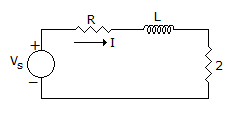A. 0.3 B. 0.4 C. 0.6 D. none

Explanation:

Vs = 10 cos ωt,

Let I be the current, then P = I2 R

I is the rms current.Prms =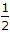I2 R4I2 = 4I = 2A.

Total power drawn =Vm Im cos θ10 x 2 cos θ = 10 cos θ

Total power cannot be determine until value of R = 12 Ω is given.

47.

The quiescent collector current IC, and collector to emitter voltage VCE in a CE connection are the values when

 A. ac signal is zero B. ac signal is low C. ac signal is negative D. either (a) or (b)

Explanation:

Quiescent-operation means operation when ac signal is not applied.

48.

In the op-amp circuit of figure, V0 =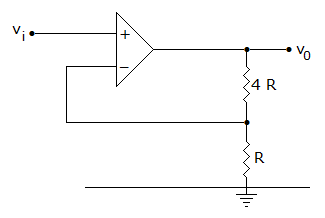A. Vi B. 3 Vi C. 5 Vi D. 0.5 Vi

Explanation: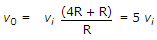.

49.

Figure shows the self bias circuit for CE amplifier and its equivalent circuit. VBB and RB respectively are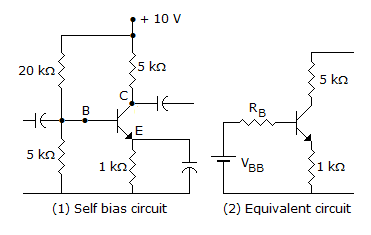A. 10 V and 4 k ohm B. 5 V and 4 k ohm C. 2 V and 4 k ohm D. 2 V and 10 k ohm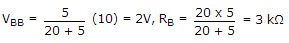.